# Types of DC Motor – Self Excited (Shunt, Series, and Compound)

Posted on

There are two general types of DC motors, a shunt and a series type.
The shunt motor has its armature and rotor coils bound in series, whereas the series motor has them in series. These configurations result in different torque characteristics.In the enclosed picture, you can see that the series motor has a high starting torque which quickly fades away, therefore the shunt motor would be more appropriate for keeping traction, no torque outbursts and constant output.

The direct current motor or the DC motor has a lot of application in today’s field of engineering and technology. Starting from an electric shaver to parts of automobiles, in all small or medium sized motoring applications DC motors come handy. And because of its wide range of application different functional types of DC motor are available in the market for specific requirements.

The types of DC motor can be listed as follows:

Now let’s do a detailed discussion about all the essential types of DC motor.

## Separately Excited DC Motor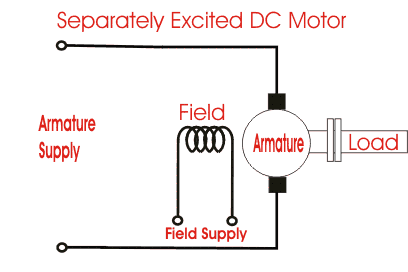As the name suggests, in case of a separately excited DC motor the supply is given separately to the field and armature windings. The main distinguishing fact in these types of DC motor is that, the armature current does not flow through the field windings, as the field winding is energized from a separate external source of DC current as shown in the figure beside.

From the torque equation of DC motor we know Tg = Ka φ Ia So the torque in this case can be varied by varying field flux φ, independent of the armature current Ia.

## Permanent Magnet DC Motor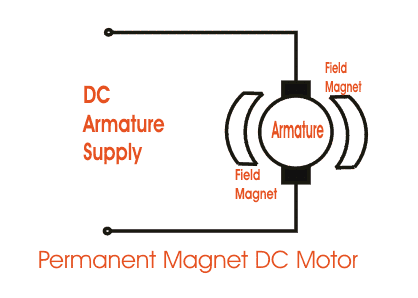The permanent magnet DC motor consists of an armature winding as in case of an usual motor, but does not necessarily contain the field windings. The construction of these types of DC motor are such that, radially magnetized permanent magnets are mounted on the inner periphery of the stator core to produce the field flux.

The rotor on the other hand has a conventional DC armature with commutator segments and brushes. The diagrammatic representation of a permanent magnet DC motor is given below. The torque equation of DC motor suggests,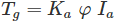Here φ is always constant, as permanent magnets of required flux density are chosen at the time of construction and can’t be changed there after. For a permanent magnet DC motor,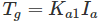Where,

Ka1 = Ka.φ which is another constant.

In this case the torque of DC Motor can only be changed by controlling armature supply.

## Self Excited DC Motor

In case of self excited DC motor, the field winding is connected either in series or in parallel or partly in series, partly in parallel to the armature winding, and on this basis its further classified as:

1. Shunt wound DC motor.
2. Series wound DC motor.
3. Compound wound DC motor.

Let’s now go into the details of these types of self excited DC motor.

### Shunt Wound DC Motor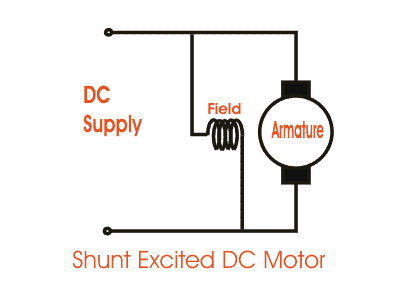In case of a shunt wound DC motor or more specifically shunt wound self excited DC motor, the field windings are exposed to the entire terminal voltage as they are connected in parallel to the armature winding as shown in the figure below. To understand the characteristic of these types of DC motor, lets consider the basic voltage equation given by,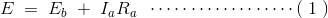[Where, E, Eb, Ia, Ra are the supply voltage, back emf, armature current and armature resistance respectively]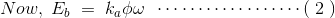[since back emf increases with flux φ and angular speed ωω] Now substituting Eb from equation (2) to equation (1) we get,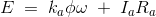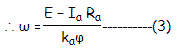The torque equation of a DC motor resembles,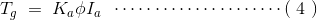This is similar to the equation of a straight line, and we can graphically representing the torque speed characteristic of a shunt wound self excited DC motor as: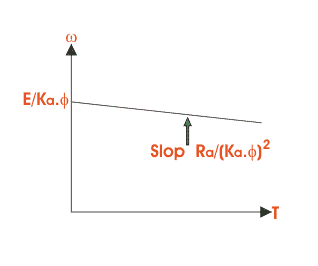The shunt wound DC motor is a constant speed motor, as the speed does not vary here with the variation of mechanical load on the output.

### Series Wound DC Motor

In case of a series wound self excited DC motor or simply series wound DC motor, the entire armature current flows through the field winding as its connected in series to the armature winding. The series wound self excited DC motor is diagrammatically represented below for clear understanding.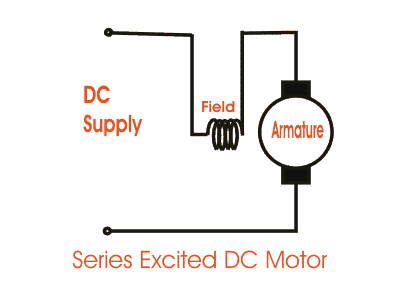Now to determint the torque speed characteristic of these types of DC motor, lets get to the torque speed equation. From the circuit diagram we can see that the voltage equation gets modified to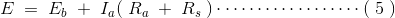Where as back emf remains Eb = kaφω Neglecting saturation we get,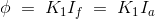[since field current = armature current]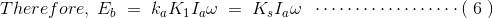From equation (5) and (6)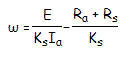From this equation we obtain the torque speed characteristic as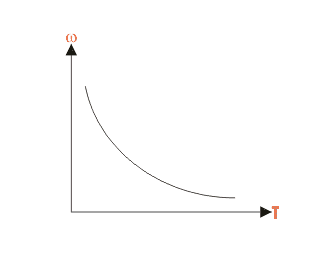In a series wound DC motor, the speed varies with load. And operation wise this is its main difference from a shunt wound DC motor.

### Compound Wound DC Motor

The compound excitation characteristic in a DC motor can be obtained by combining the operational characteristic of both the shunt and series excited DC motor. The compound wound self excited DC motor or simply compound wound DC motor essentially contains the field winding connected both in series and in parallel to the armature winding as shown in the figure below: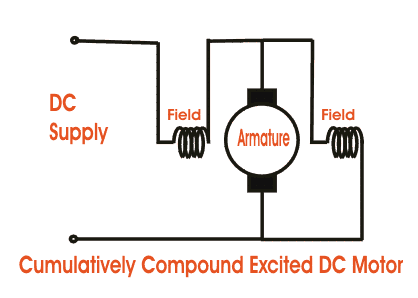The excitation of compound wound DC motor can be of two types depending on the nature of compounding.

#### Cumulative Compound DC Motor

When the shunt field flux assists the main field flux, produced by the main field connected in series to the armature winding then its called cumulative compound DC motor.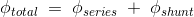#### Differential Compound DC Motor

In case of a differentially compounded self excited DC motor i.e. differential compound DC motor, the arrangement of shunt and series winding is such that the field flux produced by the shunt field winding diminishes the effect of flux by the main series field winding.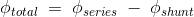The net flux produced in this case is lesser than the original flux and hence does not find much of a practical application. The compounding characteristic of the self excited DC motor is shown in the figure below.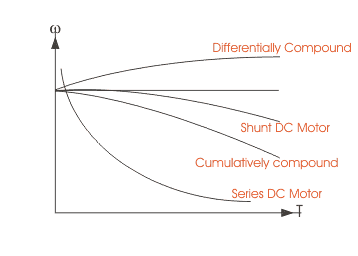Both the cumulative compound and differential compound DC motor can either be of short shunt or long shunt type depending on the nature of arrangement.

#### Short Shunt DC Motor

If the shunt field winding is only parallel to the armature winding and not the series field winding then its known as short shunt DC motor or more specifically short shunt type compound wound DC motor.

#### Long Shunt DC Motor

If the shunt field winding is parallel to both the armature winding and the series field winding then it’s known as long shunt type compounded wound DC motor or simply long shunt DC motor. Short shunt and long shunt type motors have been shown in the diagram below.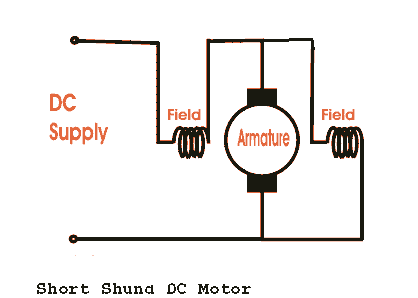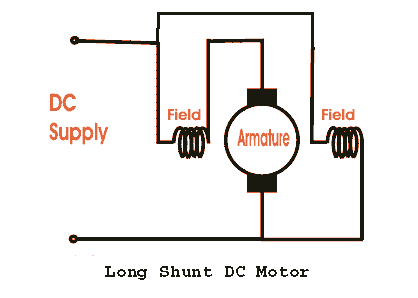### Types of DC Motor Conclusion

After going through the above portion of types of dc motor we can now establish essential types of DC motor. I hope you enjoy when reading this article, thank you.